# RD Sharma Solutions For Class 7 Maths Exercise 7.1 Chapter 7 Algebraic Expressions

RD Sharma Solutions for Class 7 Maths Exercise 7.1 of Chapter 7 Algebraic Expressions in PDF are available here. Learners can download the PDF from provided links. RD Sharma Solutions for Class 7 is the best material to refer to CBSE students. Here students will learn about the definition and meaning of algebraic expressions. Algebraic expression defined as a combination of constants and variables connected by the signs of fundamental operations of addition, subtraction, multiplication and division. Several topics of this exercise are listed below:

• Types of algebraic expressions
• Definition and meaning of factors and coefficients
• Like and unlike terms
• Finding the value of an algebraic expression

## Download the PDF of RD Sharma Solutions For Class 7 Maths Chapter 7 – Algebraic Expressions Exercise 7.1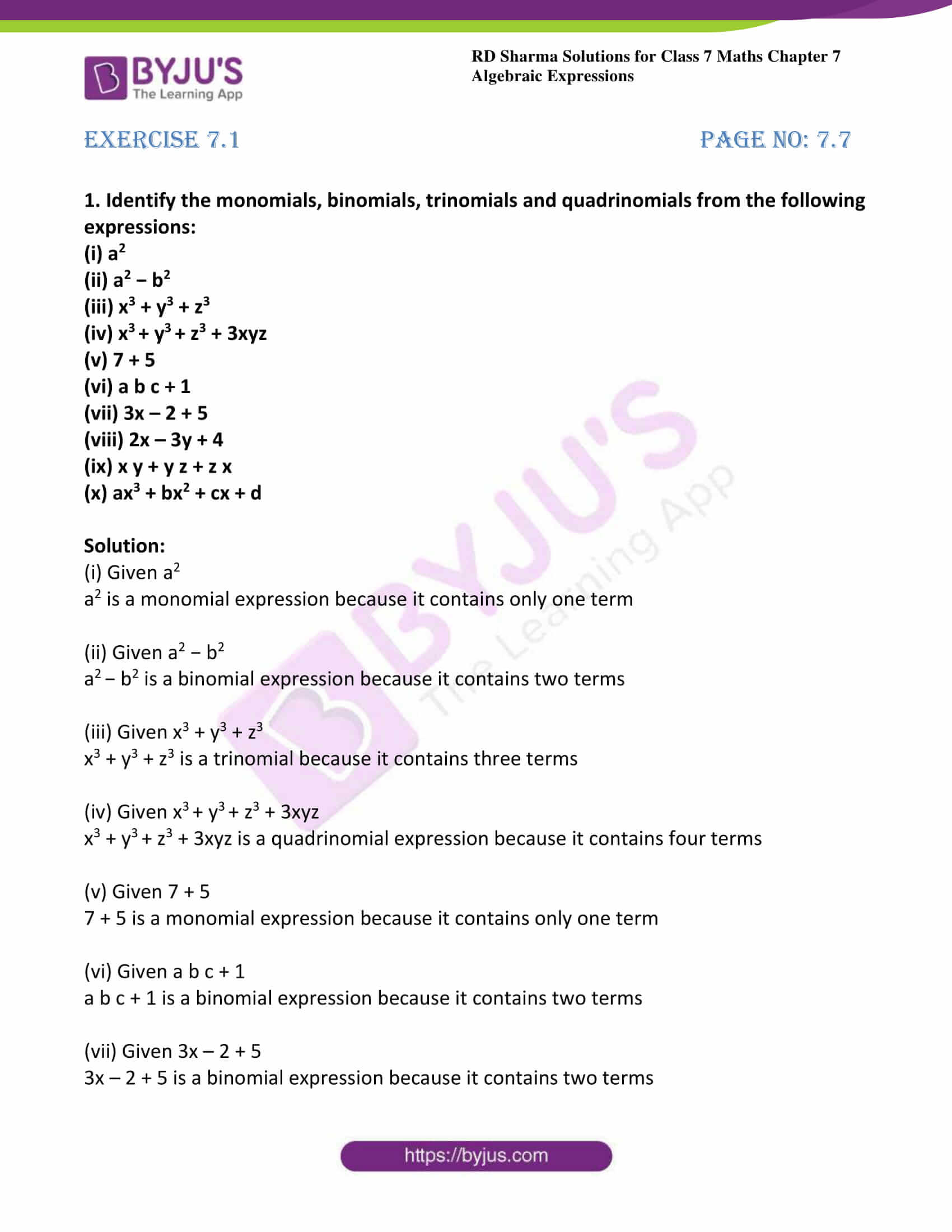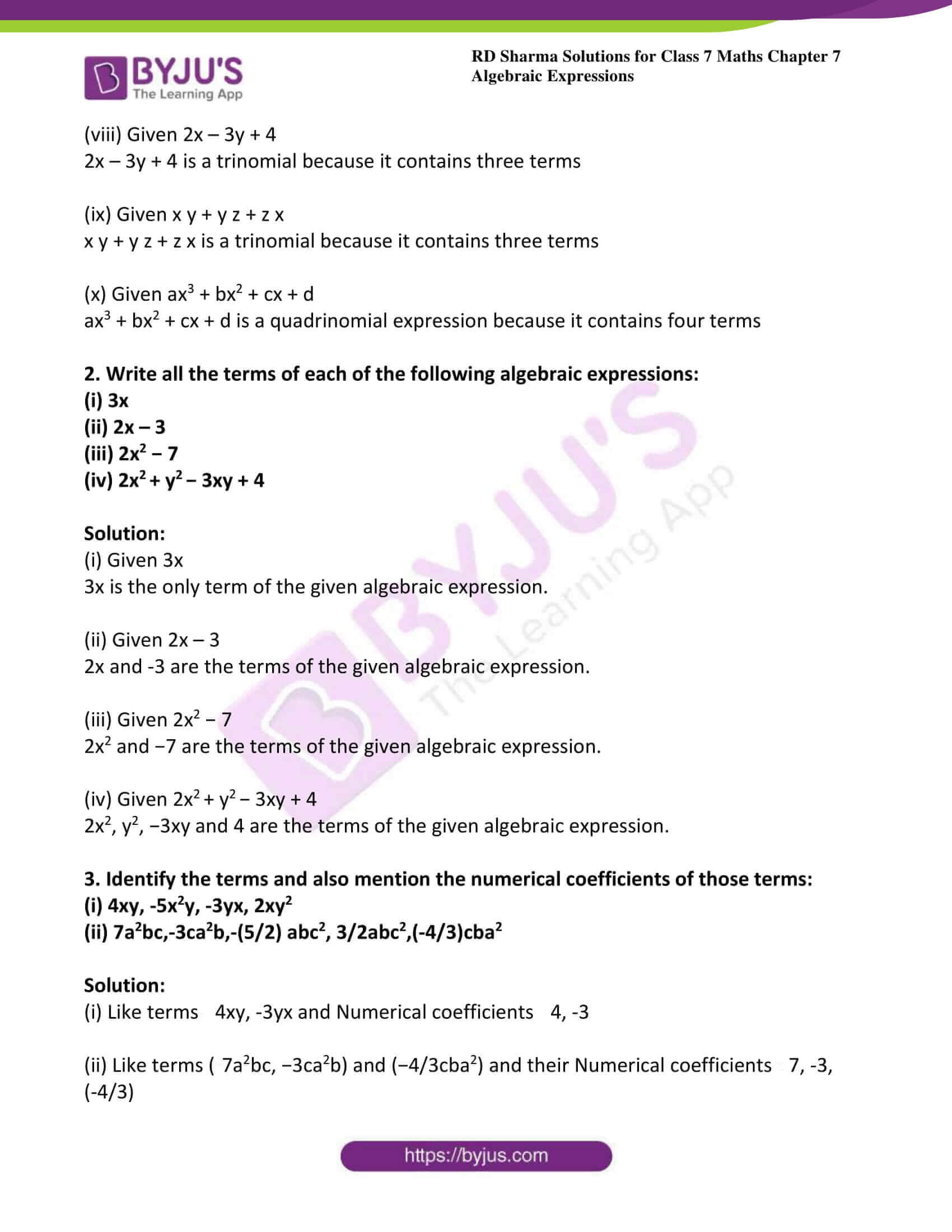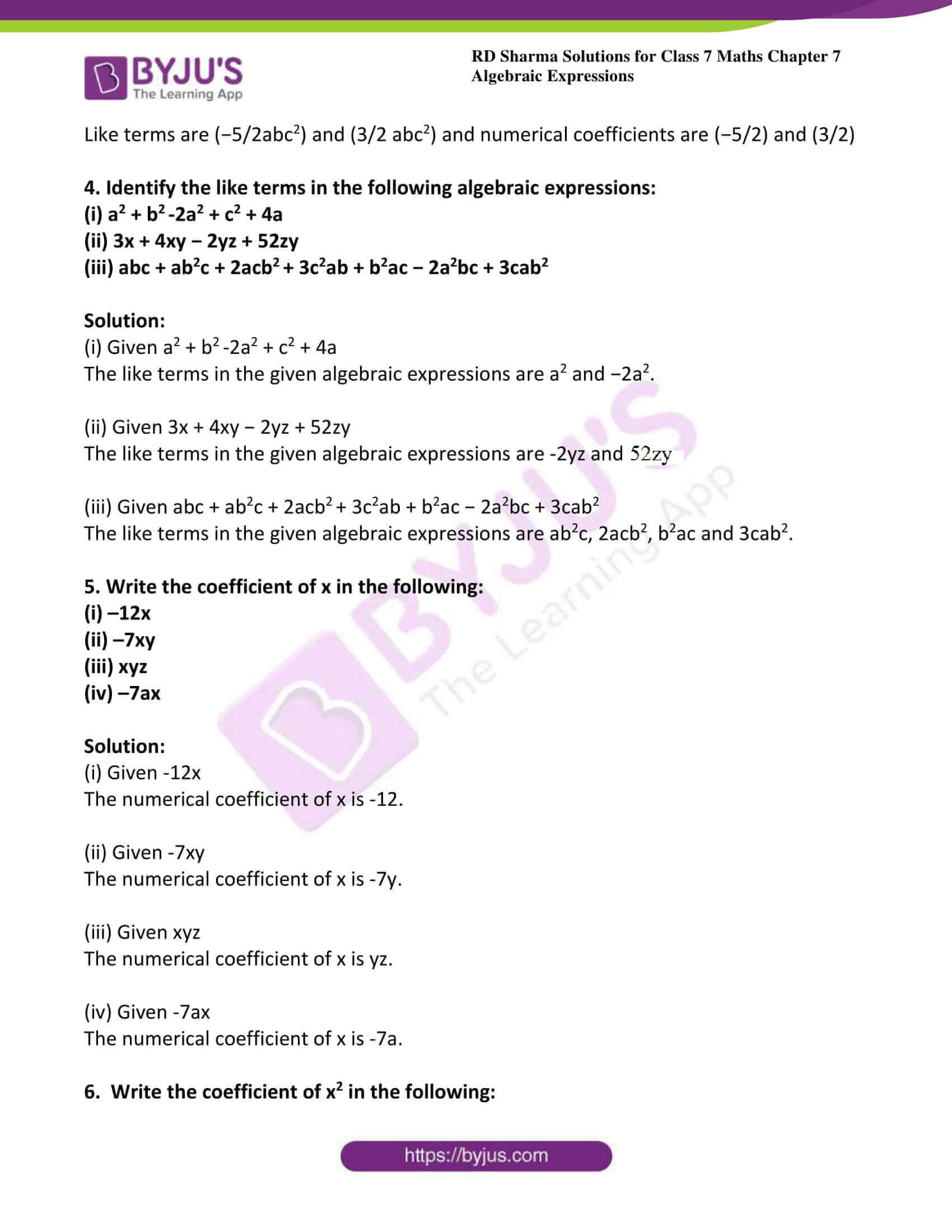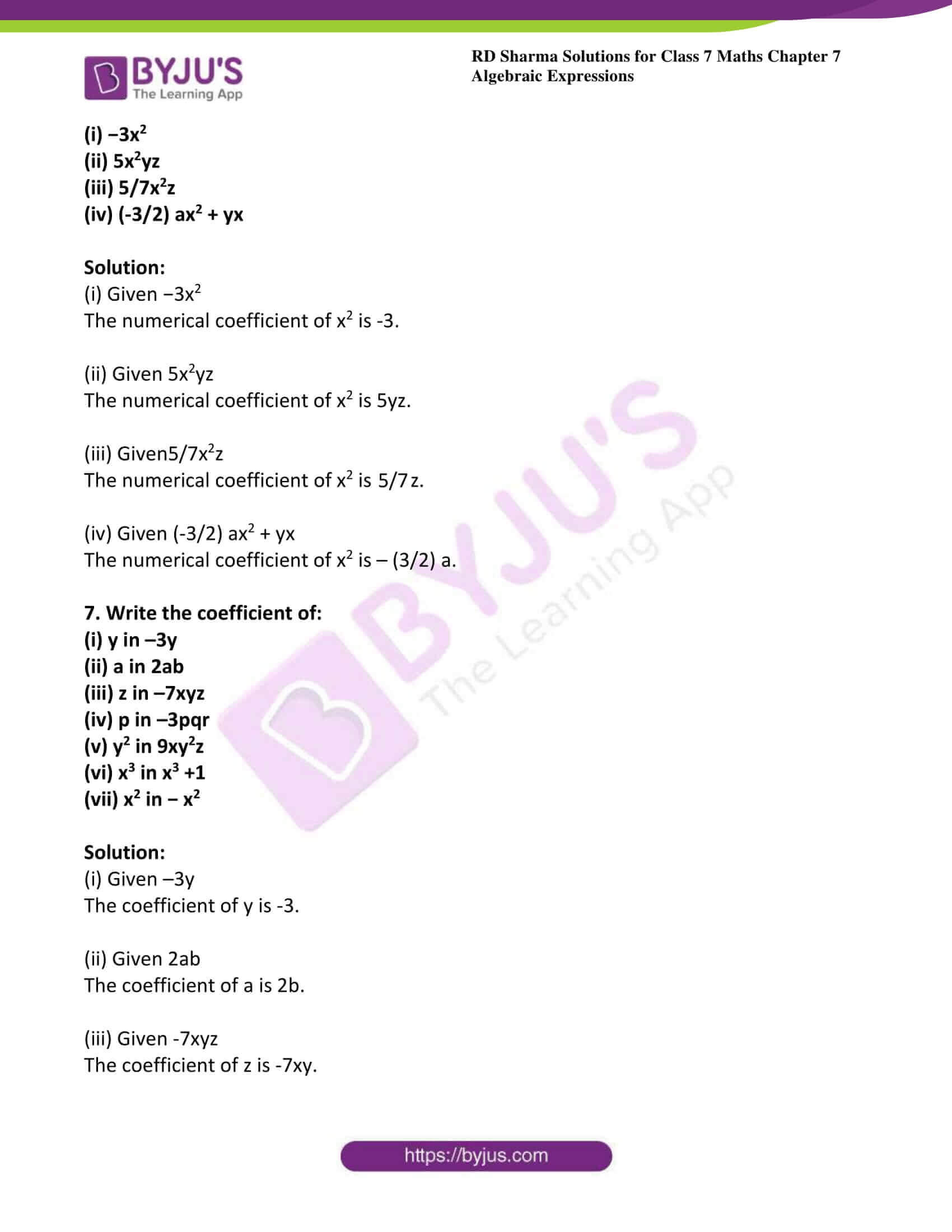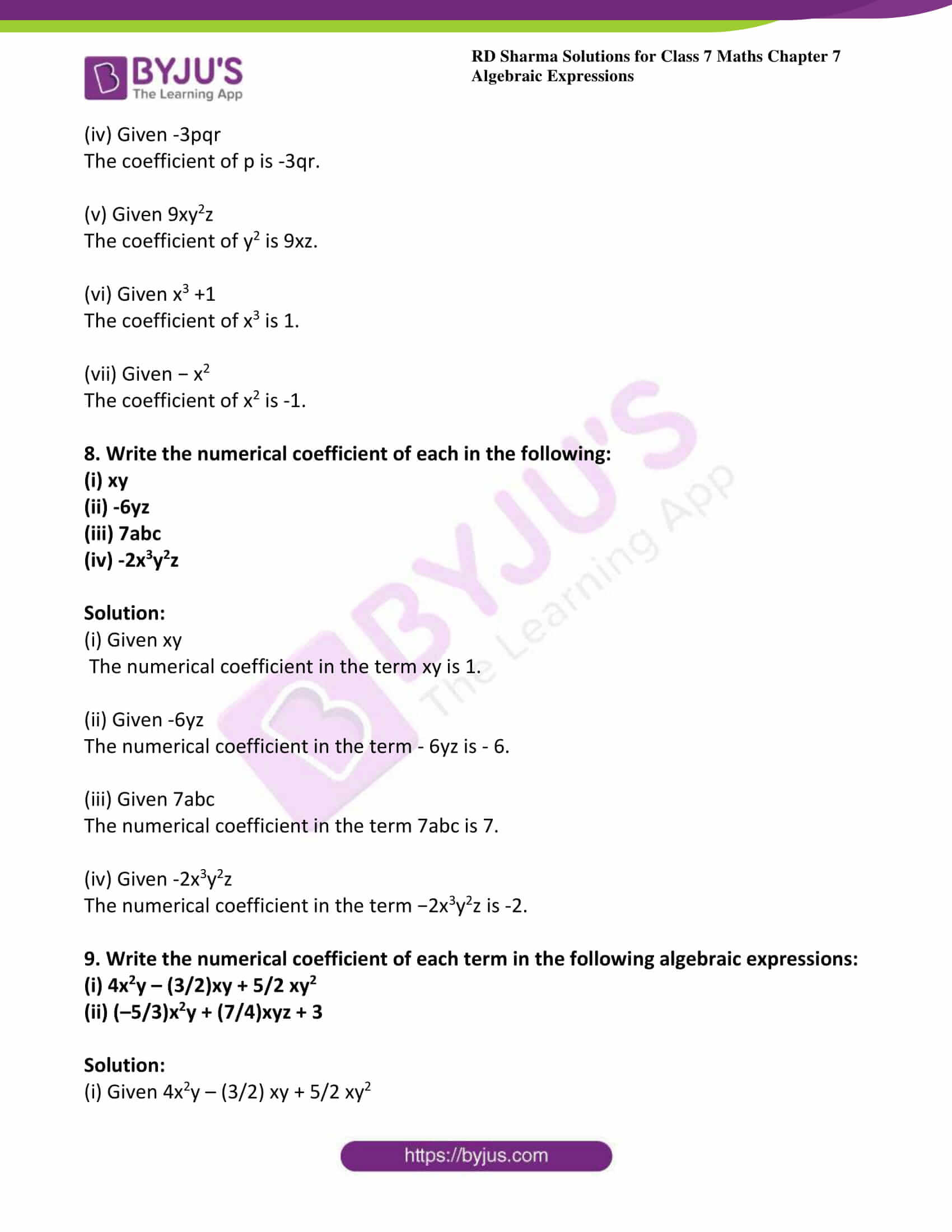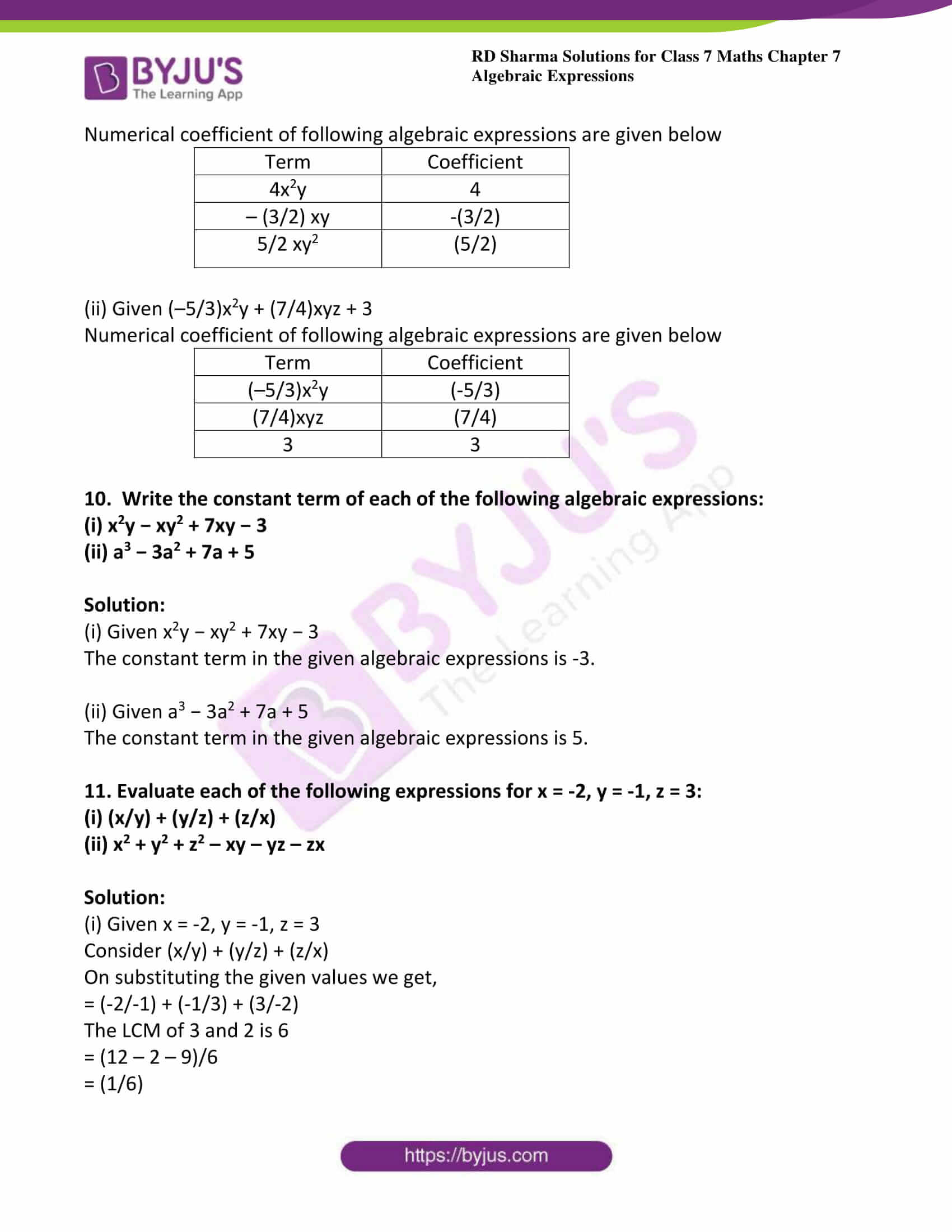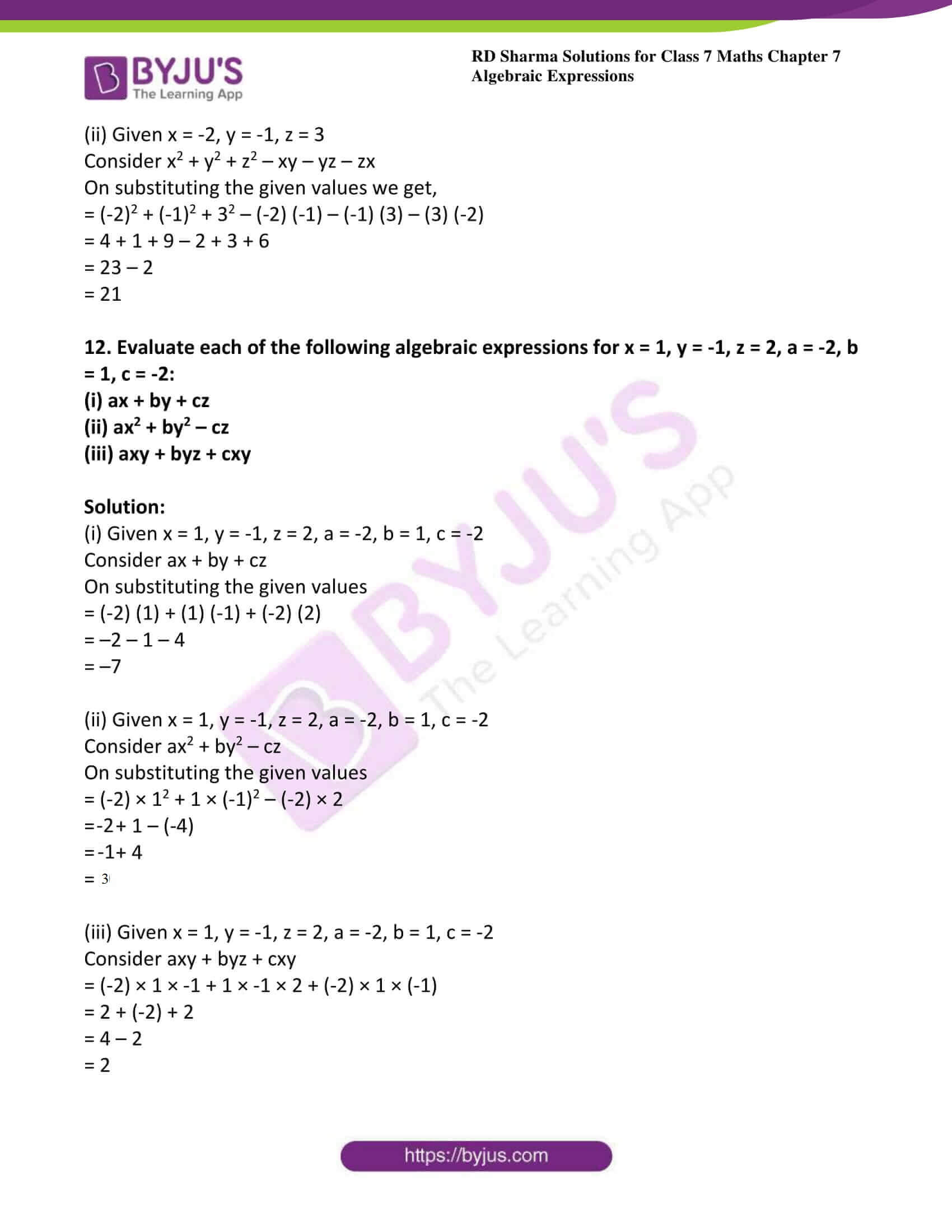### Access answers to Maths RD Sharma Solutions For Class 7 Chapter 7 – Algebraic Expressions Exercise 7.1

1. Identify the monomials, binomials, trinomials and quadrinomials from the following expressions:

(i) a2

(ii) a2 − b2

(iii) x3 + y3 + z3

(iv) x+ y+ z3 + 3xyz

(v) 7 + 5

(vi) a b c + 1

(vii) 3x – 2 + 5

(viii) 2x – 3y + 4

(ix) x y + y z + z x

(x) ax3 + bx2 + cx + d

Solution:

(i) Given a2

a2 is a monomial expression because it contains only one term

(ii) Given a2 − b2

a− b2 is a binomial expression because it contains two terms

(iii) Given x3 + y3 + z3

x3 + y3 + z3 is a trinomial because it contains three terms

(iv) Given x+ y+ z3 + 3xyz

x3 + y+ z3 + 3xyz is a quadrinomial expression because it contains four terms

(v) Given 7 + 5

7 + 5 is a monomial expression because it contains only one term

(vi) Given a b c + 1

a b c + 1 is a binomial expression because it contains two terms

(vii) Given 3x – 2 + 5

3x – 2 + 5 is a binomial expression because it contains two terms

(viii) Given 2x – 3y + 4

2x – 3y + 4 is a trinomial because it contains three terms

(ix) Given x y + y z + z x

x y + y z + z x is a trinomial because it contains three terms

(x) Given ax3 + bx2 + cx + d

ax3 + bx2 + cx + d is a quadrinomial expression because it contains four terms

2. Write all the terms of each of the following algebraic expressions:

(i) 3x

(ii) 2x – 3

(iii) 2x2 − 7

(iv) 2x+ y− 3xy + 4

Solution:

(i) Given 3x

3x is the only term of the given algebraic expression.

(ii) Given 2x – 3

2x and -3 are the terms of the given algebraic expression.

(iii) Given 2x2 − 7

2x2 and −7 are the terms of the given algebraic expression.

(iv) Given 2x+ y− 3xy + 4

2x2, y2, −3xy and 4 are the terms of the given algebraic expression.

3. Identify the terms and also mention the numerical coefficients of those terms:

(i) 4xy, -5x2y, -3yx, 2xy2

(ii) 7a2bc,-3ca2b,-(5/2) abc2, 3/2abc2,(-4/3)cba2

Solution:

(i) Like terms 4xy, -3yx and Numerical coefficients 4, -3

(ii) Like terms (7a2bc, −3ca2b) and (−4/3cba2) and their Numerical coefficients 7, -3,

(-4/3)

Like terms are (−5/2abc2) and (3/2 abc2) and numerical coefficients are (−5/2) and (3/2)

4. Identify the like terms in the following algebraic expressions:

(i) a2 + b-2a2 + c2 + 4a

(ii) 3x + 4xy − 2yz + 52zy

(iii) abc + ab2c + 2acb+ 3c2ab + b2ac − 2a2bc + 3cab2

Solution:

(i) Given a2 + b-2a2 + c2 + 4a

The like terms in the given algebraic expressions are a2 and −2a2.

(ii) Given 3x + 4xy − 2yz + 52zy

The like terms in the given algebraic expressions are -2yz and 52zy.

(iii) Given abc + ab2c + 2acb+ 3c2ab + b2ac − 2a2bc + 3cab2

The like terms in the given algebraic expressions are ab2c, 2acb2, b2ac and 3cab2.

5. Write the coefficient of x in the following:

(i) –12x

(ii) –7xy

(iii) xyz

(iv) –7ax

Solution:

(i) Given -12x

The numerical coefficient of x is -12.

(ii) Given -7xy

The numerical coefficient of x is -7y.

(iii) Given xyz

The numerical coefficient of x is yz.

(iv) Given -7ax

The numerical coefficient of x is -7a.

6. Write the coefficient of x2 in the following:

(i) −3x2

(ii) 5x2yz

(iii) 5/7x2z

(iv) (-3/2) ax2 + yx

Solution:

(i) Given −3x2

The numerical coefficient of x2 is -3.

(ii) Given 5x2yz

The numerical coefficient of x2 is 5yz.

(iii) Given 5/7x2z

The numerical coefficient of x2 is 5/7z.

(iv) Given (-3/2) ax2 + yx

The numerical coefficient of x2 is – (3/2) a.

7. Write the coefficient of:

(i) y in –3y

(ii) a in 2ab

(iii) z in –7xyz

(iv) p in –3pqr

(v) y2 in 9xy2z

(vi) x3 in x3 +1

(vii) x2 in − x2

Solution:

(i) Given –3y

The coefficient of y is -3.

(ii) Given 2ab

The coefficient of a is 2b.

(iii) Given -7xyz

The coefficient of z is -7xy.

(iv) Given -3pqr

The coefficient of p is -3qr.

(v) Given 9xy2z

The coefficient of y2 is 9xz.

(vi) Given x3 +1

The coefficient of x3 is 1.

(vii) Given − x2

The coefficient of x2 is -1.

8. Write the numerical coefficient of each in the following:

(i) xy

(ii) -6yz

(iii) 7abc

(iv) -2x3y2z

Solution:

(i) Given xy

The numerical coefficient in the term xy is 1.

(ii) Given -6yz

The numerical coefficient in the term – 6yz is – 6.

(iii) Given 7abc

The numerical coefficient in the term 7abc is 7.

(iv) Given -2x3y2z

The numerical coefficient in the term −2x3y2z is -2.

9. Write the numerical coefficient of each term in the following algebraic expressions:

(i) 4x2y – (3/2)xy + 5/2 xy2

(ii) (–5/3)x2y + (7/4)xyz + 3

Solution:

(i) Given 4x2y – (3/2) xy + 5/2 xy2

Numerical coefficient of following algebraic expressions are given below

 Term Coefficient 4x2y 4 – (3/2) xy -(3/2) 5/2 xy2 (5/2)

(ii) Given (–5/3)x2y + (7/4)xyz + 3

Numerical coefficient of following algebraic expressions are given below

 Term Coefficient (–5/3)x2y (-5/3) (7/4)xyz (7/4) 3 3

10. Write the constant term of each of the following algebraic expressions:

(i) x2y − xy2 + 7xy − 3

(ii) a3 − 3a2 + 7a + 5

Solution:

(i) Given x2y − xy2 + 7xy − 3

The constant term in the given algebraic expressions is -3.

(ii) Given a3 − 3a2 + 7a + 5

The constant term in the given algebraic expressions is 5.

11. Evaluate each of the following expressions for x = -2, y = -1, z = 3:

(i) (x/y) + (y/z) + (z/x)

(ii) x2 + y2 + z2 – xy – yz – zx

Solution:

(i) Given x = -2, y = -1, z = 3

Consider (x/y) + (y/z) + (z/x)

On substituting the given values we get,

= (-2/-1) + (-1/3) + (3/-2)

The LCM of 3 and 2 is 6

= (12 – 2 – 9)/6

= (1/6)

(ii) Given x = -2, y = -1, z = 3

Consider x2 + y2 + z2 – xy – yz – zx

On substituting the given values we get,

= (-2)2 + (-1)2 + 32 – (-2) (-1) – (-1) (3) – (3) (-2)

= 4 + 1 + 9 – 2 + 3 + 6

= 23 – 2

= 21

12. Evaluate each of the following algebraic expressions for x = 1, y = -1, z = 2, a = -2, b = 1, c = -2:

(i) ax + by + cz

(ii) ax2 + by2 – cz

(iii) axy + byz + cxy

Solution:

(i) Given x = 1, y = -1, z = 2, a = -2, b = 1, c = -2

Consider ax + by + cz

On substituting the given values

= (-2) (1) + (1) (-1) + (-2) (2)

= –2 – 1 – 4

= –7

(ii) Given x = 1, y = -1, z = 2, a = -2, b = 1, c = -2

Consider ax2 + by2 – cz

On substituting the given values

= (-2) × 12 + 1 × (-1)2 – (-2) × 2

= – 2 + 1 – (-4)

= -1 + 4

= 3

(iii) Given x = 1, y = -1, z = 2, a = -2, b = 1, c = -2

Consider axy + byz + cxy

= (-2) × 1 × -1 + 1 × -1 × 2 + (-2) × 1 × (-1)

= 2 + (-2) + 2

= 4 – 2

= 2